# Rubik’s Cube – Solving 2×2

Date:  Oct 12, 2017

I wanted to find the easiest way for Kay Kay to remember how to solve the 2×2 Rubik’s cube.  Both Jay and Kay solved it before, but I think thy had forgotten how to solve it.  Even I also forgotten how to solve it, so, I need to watch thru various Youtube videos and figure out the best way to solve it.  Not all videos teach you an easy way.  But this is in my opinion, the easiest way to solve.

I did a blog last year, but I did not detail down the steps.  Now, let’s explain in details how to do it.  So that I don’t get a complaint from Kay Kay.  Hahahaha See if he understand.  I also see Youtube, but one thing I found is there are so many solution out there.  So, the one I am presenting is actually half half solution.  What I meant by half half is half need to use your real brain to solve the white layer.  Then, the other half, use your memorising of the only two formulas and rules to solve the 2×2 Rubik’s cube in Step 2 and Step 3.

Here is the formulas:

Step 2:  R U R’ U R Ux2 R’

Step 3:  R’ F R’ Bx2 R F’ R B’x2 Rx2 Bx2

2×2 Rubik’s CubeAll you need to remember is…

1. There are six faces.
2. Opposite White face is Yellow face.
3. Opposite Green face is Blue face.
4. Opposite Red face is Orange face.
5. It is recommend to complete white face first.

Step 1:  Completing the white face.

You need to finish the white face first.  Means, you put all four white cubes together in the manner that each first layer two-cubes are the same colour.

So, to start off, locate the white cube the has the logo on it.

Observe that there this white cube has Red and Blue on it.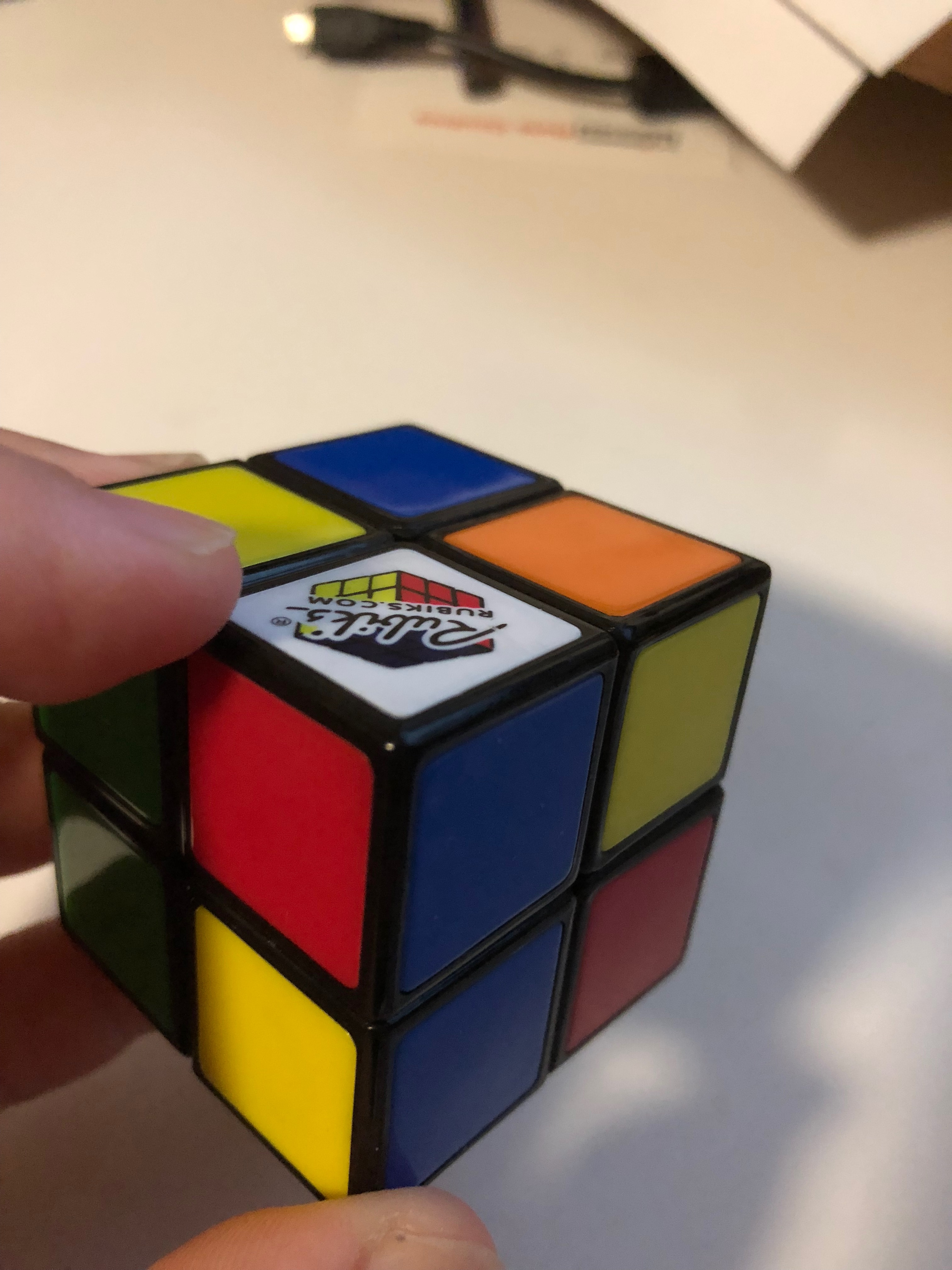Now, find ANOTHER white cube that has EITHER a red or a blue on it.  Like the one below.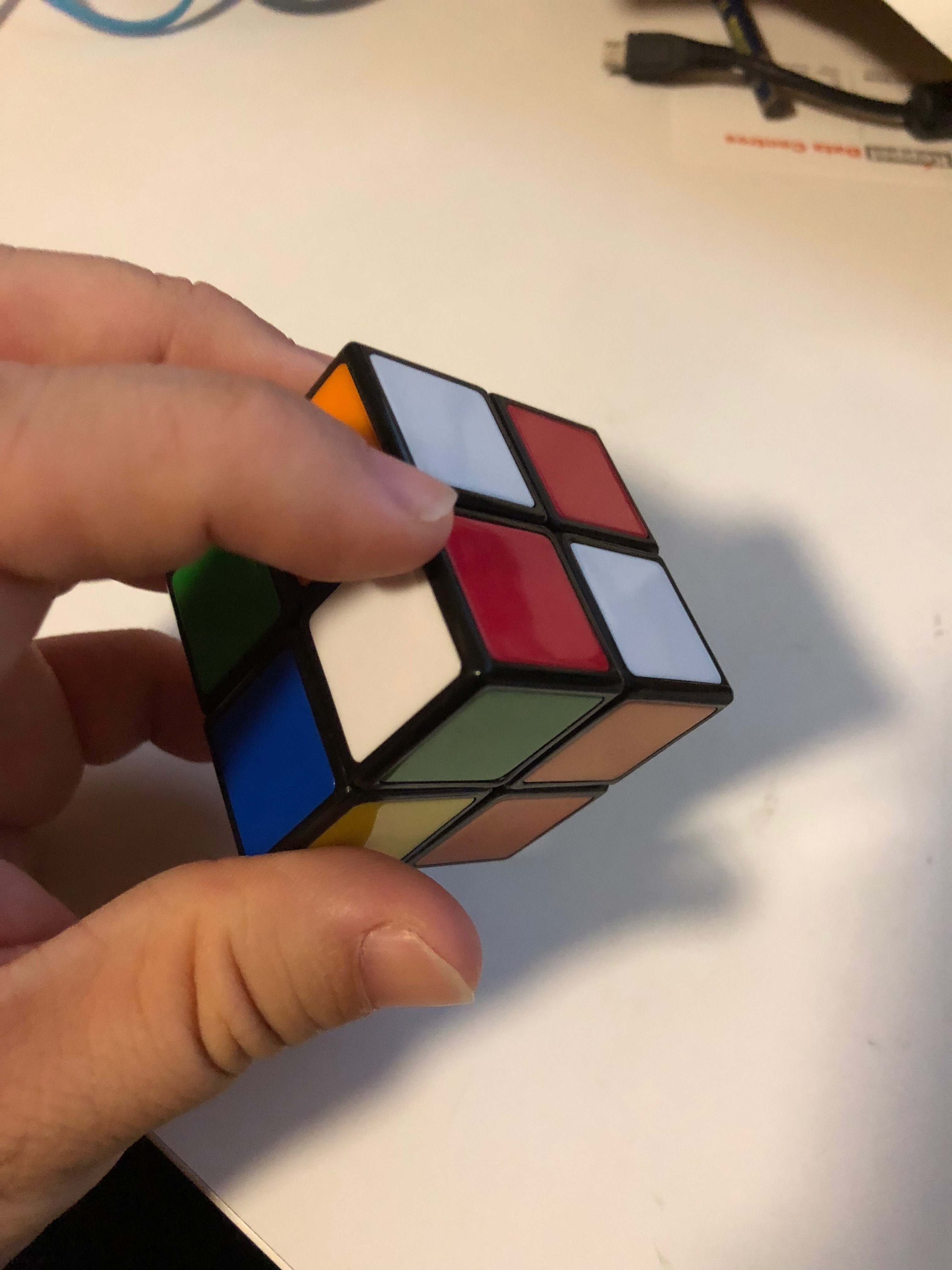So, you turn the cubes, and try to bring these two white cubes with red face come together so that both the red face are adjacent to each other.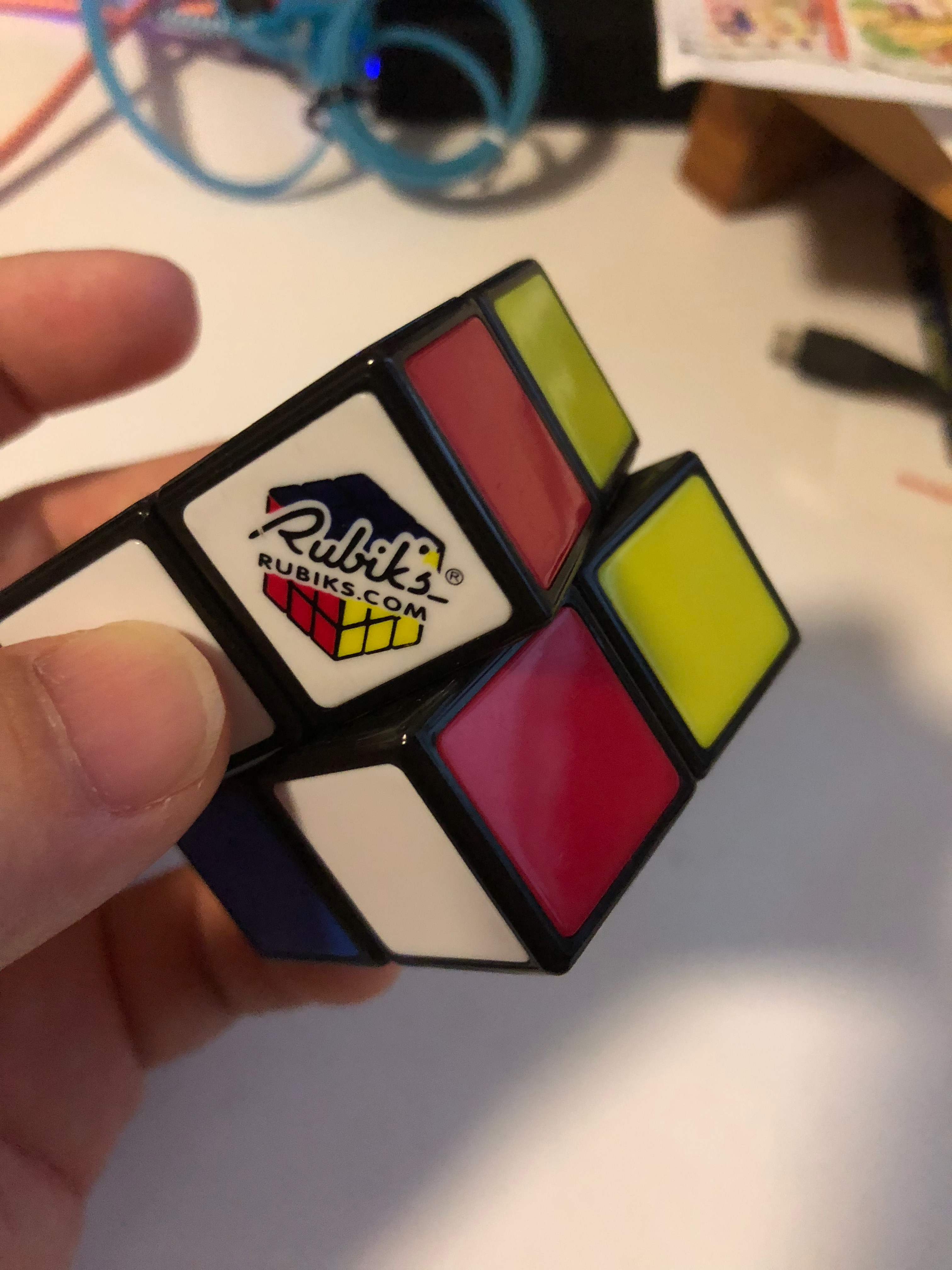Like this.

There are other people will teach you how to use Formulas to accomplish this, you don’t have to learn those formulas.  The key is to have fun in solving puzzle, and this first step is the only steps that you don’t have to use formulas.  You can solve the white face without using the formulas.  But you need to use your eyes and figure out how to turn it so to make these white cubes come together in the correct manner.So, use your head + brain, don’t use formula for Step 1 ya.  In my opinion, the key is not to solve the Rubik’s cube entirely rely on formulas.  Leave some fun for our own brain.

After you finished Step 1, you should have a complete white face, and then, adjacent to the white face, you have completed half face (upper layer) on each side.

Step 2: We will need to complete the Yellow Face (Which is opposite of White face)

Now, you will need to use formula in this stage.  The formula is:

R U R’ U R Ux2 R’

This is a formula I found from a Youtube video which I think it is easy to remember.

1. Turn the Right face clockwise
2. Turn the Upper face clockwise
3. Turn the Right face Anti-clockwise
4. Turn the Upper face clockwise (you will soon notice, the upper face is always turned clockwise)
5. Turn the Right face clockwise again
6. Turn the Upper face clockwise TWO (2) times
7. Turn the Right face Anti-clockwise

You see what I mean?  It is only turning the right hand side, in an orderly order, clockwise then anti-clockwise then clockwise again, and lastly anti-clockwise.  Every time you turn the Right face, either clockwise, or anti-clockwise, you must make the upper face turn in the same direction.  And only on the third right face turn, you turn the upper face two (2) times.How do you use this formula?

1. You must position your 2×2 Rubik’s cube in such a way that white face is facing down.  That means, your Yellow face needed to be solved is facing upside.
2. There is no fixed number of times you need to use this formula.  You will need to repeat to use this formula until you solve four yellow cubes in one face.  The adjacent cooler of the yellow cubes can be random, no need to be in pairs too.
3. Every time you finish turning using this formula, you must observe the cube, so that the cube is re-oriented to be in the following 3 scenarios.  Every time, you must turn your cube and oriented in this preferred orientation.
• If you have none yellow cube on the top face.  Then, move the cube in such a way that there is this single yellow cube facing you.  As in the following picture, there is no yellow on the top face, so, you turn the Rubik’s cube so that there is a single yellow cube on the right facing you.  (not on the left)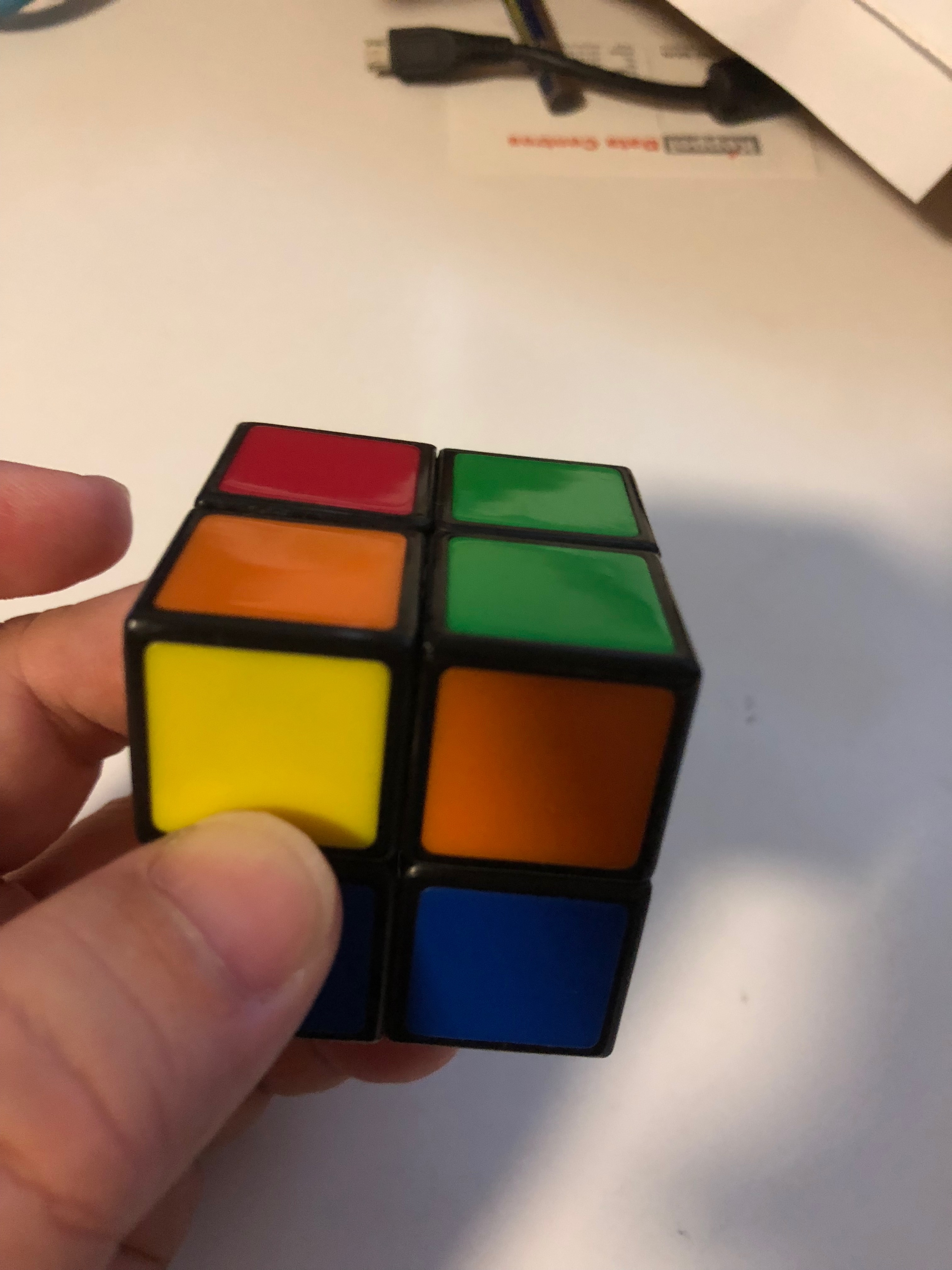• If you end up seeing two yellow cubes on the top face sitting side by side, then, you need to orient the cube in such a way that those two yellow cube adjacent to each other is facing up and at the upper layer on the top face.   As shown in the picture.• If you end up with a single yellow cube (or two yellow cubes) on the top face, then, you always orient the cube so that the single yellow cube or one of the two yellow cubes are at the bottom right corner as shown.You might end up using this formula multiple times.  1-6 times or even more times.  But eventually, you will end up with this.

Let’s recap.  In this Step 2, you will need to orient the cube in one of the three (3) position before you use the Step 2 formula.  After applying the formula, you have to re-orient the cube so that it has either the two cubes are in the top upper layer, or one of the single cube on the top face is at the right bottom corner or if there is no yellow cubes on the top face, you orient the cube so that one single yellow cube is on the top right corner on the face facing you.  Apply the same formula multiple times, each time re-orient the cube into the mentioned orientation, then, eventually, you get  here.  All four yellow cubes are together on the top face.You have solved the yellow top face with white face completely solve at the bottom.

Step 3:  Apply the Step 3 formula, re-orient a completed face (Red / Green / Blue or Orange) at the Back Face and re-apply the formula.

You need the step 3 formula in this step.

R’ F R’ B’x2 R F’ R B’x2 Rx2 B’x2

Now, if you look at it, the B’x2 is actually the same as Bx2.  Depends on how you want to remember it, and which direction of turning is more suitable for your hand.  It is up to you.  So, you can easily change it to

R’ F R’ Bx2 R F’ R Bx2 Rx2 Bx2

or even

R’ F R’ Bx2 R F’ R B’x2 Rx2 Bx2     —-> Preferred

This is how I remember it.  You need to do a set of R’ (Reverse Right hand plane) two times.  So, R’ follow by Front F, R’ follow by Bx2 (turn two times on the back plane).  Then , you do completely opposite set.  R follow by reverse Front F, R follow by B’x2, and always finished it with a Rx2 and Bx2.

Easy to remember.  Sometimes if you watch the Youtube, it might confuse you, so, what I tried to do here is to try to make it easier to remember.1. After you have just solved the top yellow face.  You end up with either of the two scenario.
2. Scenario 1, you do not have another completed face on the side.  So, you simply run the formula one time, with the yellow face point up, white face point down.
3. Scenario 2, you have a completed face on the side.  Like this one shown in the photo.  I have another blue face completed.
4. Please take note, after you have apply the step 3 formula, you might need to adjust the top layer so that you can have a compete blue face (or red face or orange face or green face).
5. Keep on apply Step 3 formula, but before you do the Step 3 formula, you must point that completed face (in our example, blue face) towards the back.  i.e. Blue face face the back (away from you) and then you re-do the Step 3 formula.I think you just have to apply the Step 3 formula 2-3 times, you will solve your 2×2 Rubik’s Cube.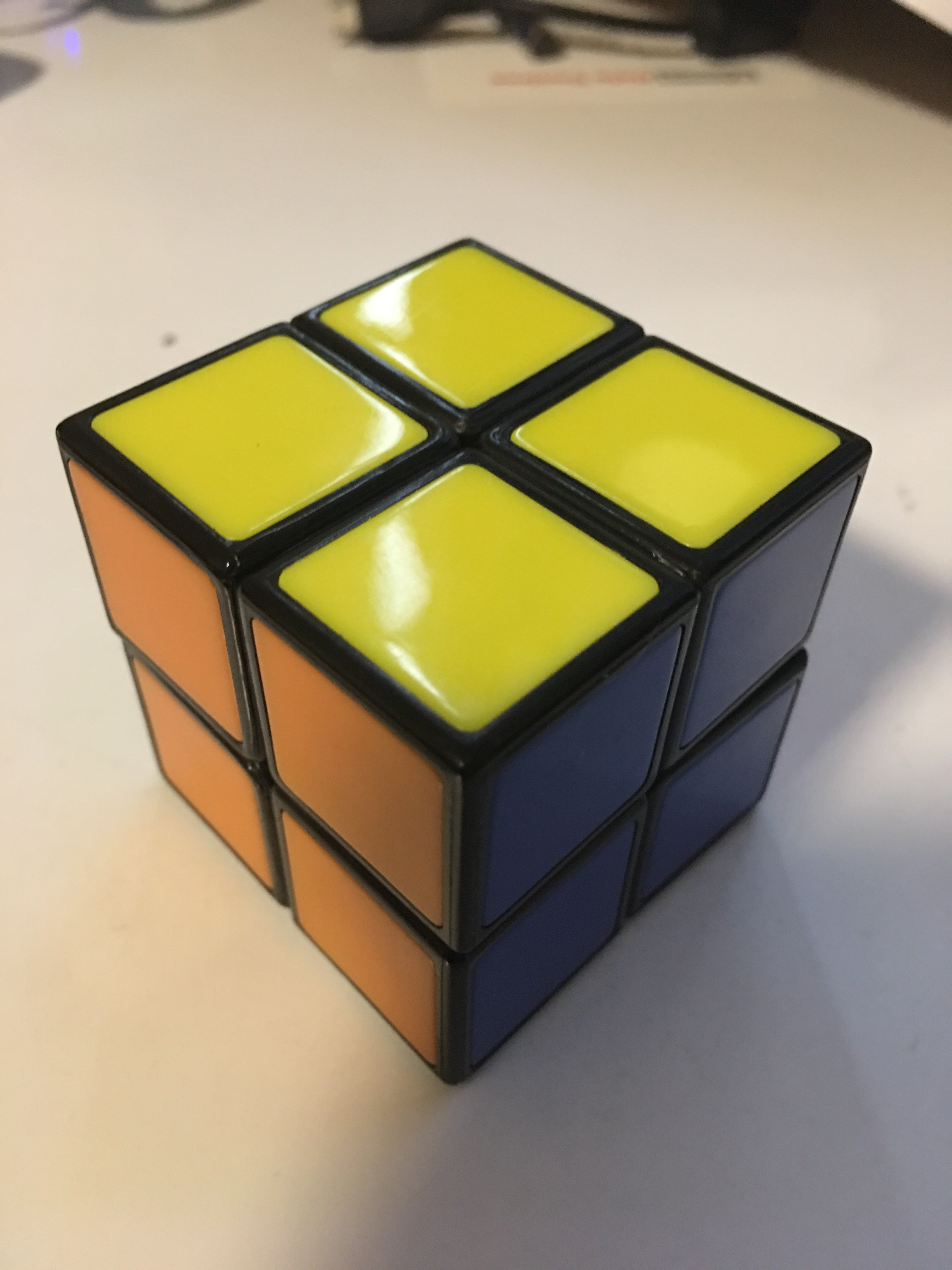Remember, every time after you apply Step 3 formula, you need to re-arrange your top layer.  Just turn it around to find a complete face or to solve the puzzle.  Yeah.

I randomise my cute and reset it.  And try to show you in a complete tutorial how to solve it.

Lastly to end this tutorial, can you easily do the following?  Each face of the six faces, has four different colour.  Hahaha